# Causes of change

28 Mar 2012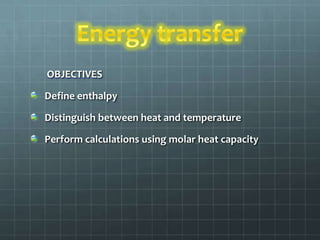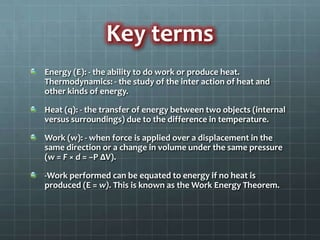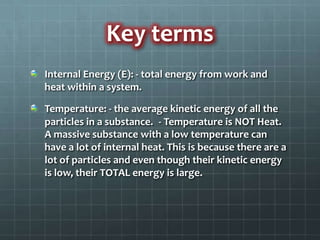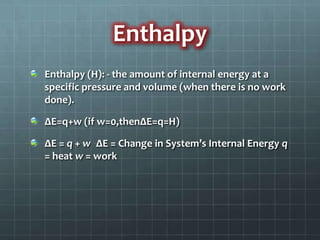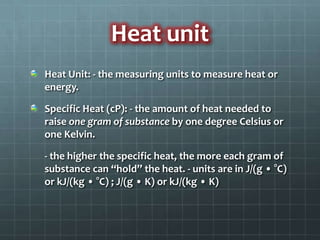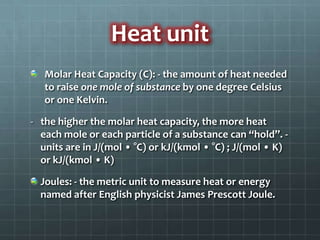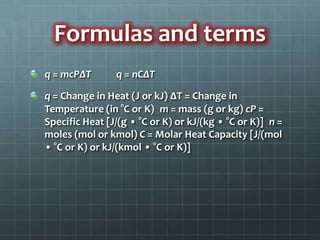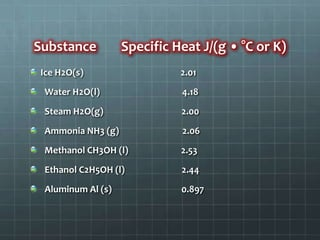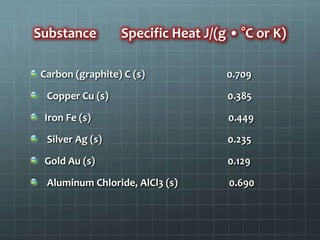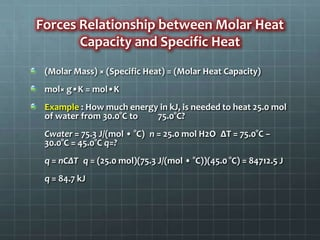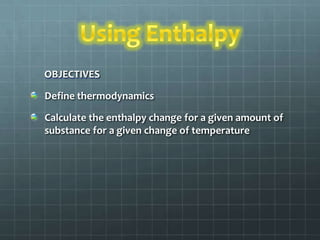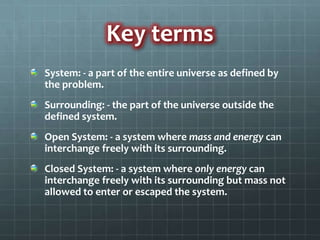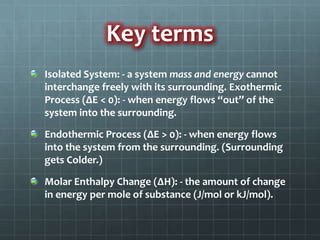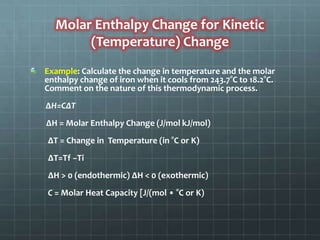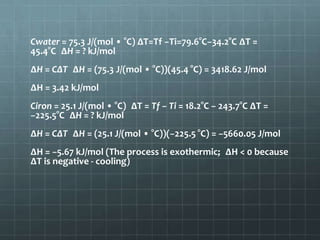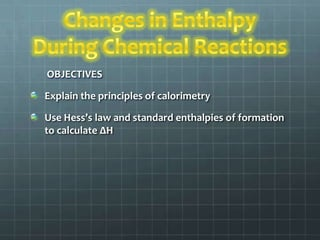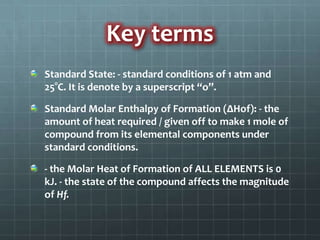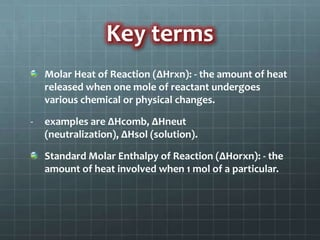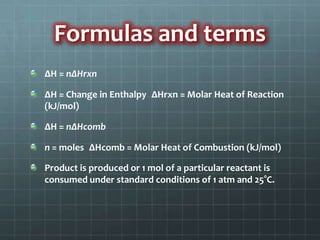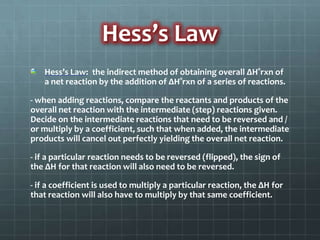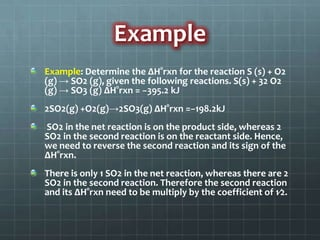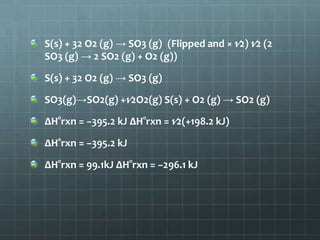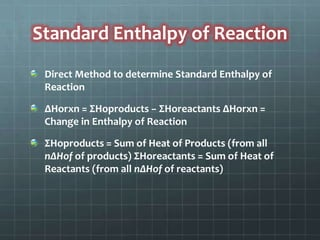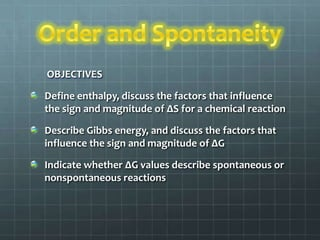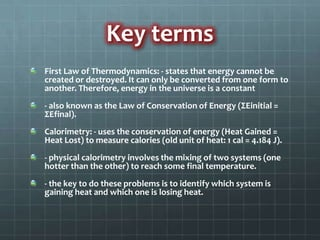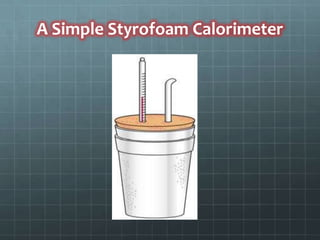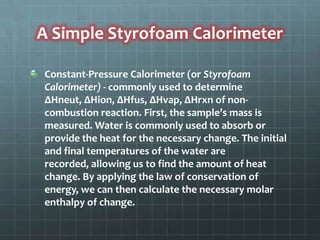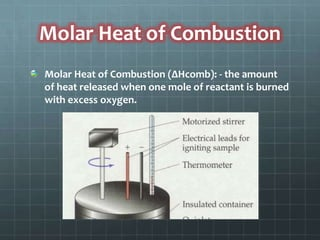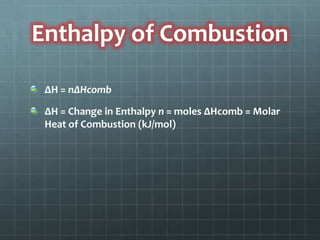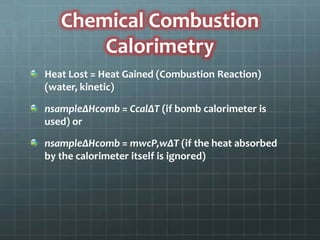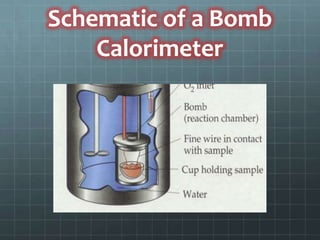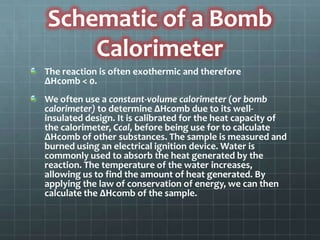1 sur 34

### Causes of change

• 2. OBJECTIVES Define enthalpy Distinguish between heat and temperature Perform calculations using molar heat capacity
• 3. Key terms Energy (E): - the ability to do work or produce heat. Thermodynamics: - the study of the inter action of heat and other kinds of energy. Heat (q): - the transfer of energy between two objects (internal versus surroundings) due to the difference in temperature. Work (w): - when force is applied over a displacement in the same direction or a change in volume under the same pressure (w = F × d = −P ΔV). -Work performed can be equated to energy if no heat is produced (E = w). This is known as the Work Energy Theorem.
• 4. Key terms Internal Energy (E): - total energy from work and heat within a system. Temperature: - the average kinetic energy of all the particles in a substance. - Temperature is NOT Heat. A massive substance with a low temperature can have a lot of internal heat. This is because there are a lot of particles and even though their kinetic energy is low, their TOTAL energy is large.
• 5. Enthalpy Enthalpy (H): - the amount of internal energy at a specific pressure and volume (when there is no work done). ΔE=q+w (if w=0,thenΔE=q=H) ΔE = q + w ΔE = Change in System’s Internal Energy q = heat w = work
• 6. Heat unit Heat Unit: - the measuring units to measure heat or energy. Specific Heat (cP): - the amount of heat needed to raise one gram of substance by one degree Celsius or one Kelvin. - the higher the specific heat, the more each gram of substance can “hold” the heat. - units are in J/(g • °C) or kJ/(kg • °C) ; J/(g • K) or kJ/(kg • K)
• 7. Heat unit Molar Heat Capacity (C): - the amount of heat needed to raise one mole of substance by one degree Celsius or one Kelvin. - the higher the molar heat capacity, the more heat each mole or each particle of a substance can “hold”. - units are in J/(mol • °C) or kJ/(kmol • °C) ; J/(mol • K) or kJ/(kmol • K) Joules: - the metric unit to measure heat or energy named after English physicist James Prescott Joule.
• 8. Formulas and terms q = mcPΔT q = nCΔT q = Change in Heat (J or kJ) ΔT = Change in Temperature (in °C or K) m = mass (g or kg) cP = Specific Heat [J/(g • °C or K) or kJ/(kg • °C or K)] n = moles (mol or kmol) C = Molar Heat Capacity [J/(mol • °C or K) or kJ/(kmol • °C or K)]
• 9. Substance Specific Heat J/(g • °C or K) Ice H2O(s) 2.01 Water H2O(l) 4.18 Steam H2O(g) 2.00 Ammonia NH3 (g) 2.06 Methanol CH3OH (l) 2.53 Ethanol C2H5OH (l) 2.44 Aluminum Al (s) 0.897
• 10. Substance Specific Heat J/(g • °C or K) Carbon (graphite) C (s) 0.709 Copper Cu (s) 0.385 Iron Fe (s) 0.449 Silver Ag (s) 0.235 Gold Au (s) 0.129 Aluminum Chloride, AlCl3 (s) 0.690
• 11. Forces Relationship between Molar Heat Capacity and Specific Heat (Molar Mass) × (Specific Heat) = (Molar Heat Capacity) mol× g•K = mol•K Example : How much energy in kJ, is needed to heat 25.0 mol of water from 30.0°C to 75.0°C? Cwater = 75.3 J/(mol • °C) n = 25.0 mol H2O ΔT = 75.0°C − 30.0°C = 45.0°C q=? q = nCΔT q = (25.0 mol)(75.3 J/(mol • °C))(45.0 °C) = 84712.5 J q = 84.7 kJ
• 12. OBJECTIVES Define thermodynamics Calculate the enthalpy change for a given amount of substance for a given change of temperature
• 13. Key terms System: - a part of the entire universe as defined by the problem. Surrounding: - the part of the universe outside the defined system. Open System: - a system where mass and energy can interchange freely with its surrounding. Closed System: - a system where only energy can interchange freely with its surrounding but mass not allowed to enter or escaped the system.
• 14. Key terms Isolated System: - a system mass and energy cannot interchange freely with its surrounding. Exothermic Process (ΔE < 0): - when energy flows “out” of the system into the surrounding. Endothermic Process (ΔE > 0): - when energy flows into the system from the surrounding. (Surrounding gets Colder.) Molar Enthalpy Change (∆H): - the amount of change in energy per mole of substance (J/mol or kJ/mol).
• 15. Molar Enthalpy Change for Kinetic (Temperature) Change Example: Calculate the change in temperature and the molar enthalpy change of iron when it cools from 243.7°C to 18.2°C. Comment on the nature of this thermodynamic process. ∆H=CΔT ∆H = Molar Enthalpy Change (J/mol kJ/mol) ΔT = Change in Temperature (in °C or K) ∆T=Tf −Ti ∆H > 0 (endothermic) ∆H < 0 (exothermic) C = Molar Heat Capacity [J/(mol • °C or K)
• 16. Cwater = 75.3 J/(mol • °C) ΔT=Tf −Ti=79.6°C−34.2°C ∆T = 45.4°C ∆H = ? kJ/mol ∆H = CΔT ∆H = (75.3 J/(mol • °C))(45.4 °C) = 3418.62 J/mol ∆H = 3.42 kJ/mol Ciron = 25.1 J/(mol • °C) ΔT = Tf − Ti = 18.2°C − 243.7°C ∆T = −225.5°C ∆H = ? kJ/mol ∆H = CΔT ∆H = (25.1 J/(mol • °C))(−225.5 °C) = −5660.05 J/mol ∆H = −5.67 kJ/mol (The process is exothermic; ∆H < 0 because ∆T is negative - cooling)
• 17. OBJECTIVES Explain the principles of calorimetry Use Hess’s law and standard enthalpies of formation to calculate ΔH
• 18. Key terms Standard State: - standard conditions of 1 atm and 25°C. It is denote by a superscript “o”. Standard Molar Enthalpy of Formation (ΔHof): - the amount of heat required / given off to make 1 mole of compound from its elemental components under standard conditions. - the Molar Heat of Formation of ALL ELEMENTS is 0 kJ. - the state of the compound affects the magnitude of Hf.
• 19. Key terms Molar Heat of Reaction (ΔHrxn): - the amount of heat released when one mole of reactant undergoes various chemical or physical changes. - examples are ΔHcomb, ΔHneut (neutralization), ΔHsol (solution). Standard Molar Enthalpy of Reaction (ΔHorxn): - the amount of heat involved when 1 mol of a particular.
• 20. Formulas and terms ΔH = nΔHrxn ΔH = Change in Enthalpy ΔHrxn = Molar Heat of Reaction (kJ/mol) ΔH = nΔHcomb n = moles ΔHcomb = Molar Heat of Combustion (kJ/mol) Product is produced or 1 mol of a particular reactant is consumed under standard conditions of 1 atm and 25°C.
• 21. Hess’s Law Hess’s Law: the indirect method of obtaining overall ΔH°rxn of a net reaction by the addition of ΔH°rxn of a series of reactions. - when adding reactions, compare the reactants and products of the overall net reaction with the intermediate (step) reactions given. Decide on the intermediate reactions that need to be reversed and / or multiply by a coefficient, such that when added, the intermediate products will cancel out perfectly yielding the overall net reaction. - if a particular reaction needs to be reversed (flipped), the sign of the ΔH for that reaction will also need to be reversed. - if a coefficient is used to multiply a particular reaction, the ΔH for that reaction will also have to multiply by that same coefficient.
• 22. Example Example: Determine the ΔH°rxn for the reaction S (s) + O2 (g) → SO2 (g), given the following reactions. S(s) + 32 O2 (g) → SO3 (g) ΔH°rxn = −395.2 kJ 2SO2(g) +O2(g)→2SO3(g) ΔH°rxn =−198.2kJ SO2 in the net reaction is on the product side, whereas 2 SO2 in the second reaction is on the reactant side. Hence, we need to reverse the second reaction and its sign of the ΔH°rxn. There is only 1 SO2 in the net reaction, whereas there are 2 SO2 in the second reaction. Therefore the second reaction and its ΔH°rxn need to be multiply by the coefficient of 1⁄2.
• 23. S(s) + 32 O2 (g) → SO3 (g) (Flipped and × 1⁄2) 1⁄2 (2 SO3 (g) → 2 SO2 (g) + O2 (g)) S(s) + 32 O2 (g) → SO3 (g) SO3(g)→SO2(g) +1⁄2O2(g) S(s) + O2 (g) → SO2 (g) ΔH°rxn = −395.2 kJ ΔH°rxn = 1⁄2(+198.2 kJ) ΔH°rxn = −395.2 kJ ΔH°rxn = 99.1kJ ΔH°rxn = −296.1 kJ
• 24. Standard Enthalpy of Reaction Direct Method to determine Standard Enthalpy of Reaction ΔHorxn = ΣHoproducts − ΣHoreactants ΔHorxn = Change in Enthalpy of Reaction ΣHoproducts = Sum of Heat of Products (from all nΔHof of products) ΣHoreactants = Sum of Heat of Reactants (from all nΔHof of reactants)
• 25. OBJECTIVES Define enthalpy, discuss the factors that influence the sign and magnitude of ΔS for a chemical reaction Describe Gibbs energy, and discuss the factors that influence the sign and magnitude of ΔG Indicate whether ΔG values describe spontaneous or nonspontaneous reactions
• 26. Key terms First Law of Thermodynamics: - states that energy cannot be created or destroyed. It can only be converted from one form to another. Therefore, energy in the universe is a constant - also known as the Law of Conservation of Energy (ΣEinitial = ΣEfinal). Calorimetry: - uses the conservation of energy (Heat Gained = Heat Lost) to measure calories (old unit of heat: 1 cal = 4.184 J). - physical calorimetry involves the mixing of two systems (one hotter than the other) to reach some final temperature. - the key to do these problems is to identify which system is gaining heat and which one is losing heat.
• 27. A Simple Styrofoam Calorimeter
• 28. A Simple Styrofoam Calorimeter Constant-Pressure Calorimeter (or Styrofoam Calorimeter) - commonly used to determine ΔHneut, ΔHion, ΔHfus, ΔHvap, ΔHrxn of non- combustion reaction. First, the sample’s mass is measured. Water is commonly used to absorb or provide the heat for the necessary change. The initial and final temperatures of the water are recorded, allowing us to find the amount of heat change. By applying the law of conservation of energy, we can then calculate the necessary molar enthalpy of change.
• 29. Molar Heat of Combustion Molar Heat of Combustion (ΔHcomb): - the amount of heat released when one mole of reactant is burned with excess oxygen.
• 30. Enthalpy of Combustion ΔH = nΔHcomb ΔH = Change in Enthalpy n = moles ΔHcomb = Molar Heat of Combustion (kJ/mol)
• 31. Chemical Combustion Calorimetry Heat Lost = Heat Gained (Combustion Reaction) (water, kinetic) nsampleΔHcomb = CcalΔT (if bomb calorimeter is used) or nsampleΔHcomb = mwcP,wΔT (if the heat absorbed by the calorimeter itself is ignored)
• 32. Schematic of a Bomb Calorimeter
• 33. Schematic of a Bomb Calorimeter The reaction is often exothermic and therefore ΔHcomb < 0. We often use a constant-volume calorimeter (or bomb calorimeter) to determine ΔHcomb due to its well- insulated design. It is calibrated for the heat capacity of the calorimeter, Ccal, before being use for to calculate ΔHcomb of other substances. The sample is measured and burned using an electrical ignition device. Water is commonly used to absorb the heat generated by the reaction. The temperature of the water increases, allowing us to find the amount of heat generated. By applying the law of conservation of energy, we can then calculate the ΔHcomb of the sample.
• 34. The world is made up of chemical reactions, it’s the way how you look at it and take it to your stride